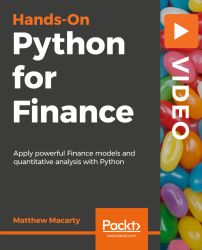•#### Hands-On Python for Finance [Video]#### Overview of this book

Did you know Python is the one of the best solution to quantitatively analyse your finances by taking an overview of your timeline? This hands-on course helps both developers and quantitative analysts to get started with Python, and guides you through the most important aspects of using Python for quantitative finance. You will begin with a primer to Python and its various data structures.Then you will dive into third party libraries. You will work with Python libraries and tools designed specifically for analytical and visualization purposes. Then you will get an overview of cash flow across the timeline. You will also learn concepts like Time Series Evaluation, Forecasting, Linear Regression and also look at crucial aspects like Linear Models, Correlation and portfolio construction. Finally, you will compute Value at Risk (VaR) and simulate portfolio values using Monte Carlo Simulation which is a broader class of computational algorithms. With numerous practical examples through the course, you will develop a full-fledged framework for Monte Carlo, which is a class of computational algorithms and simulation-based derivatives and risk analytics. The Github Link to this course is: https://github.com/PacktPublishing/Hands-on-Python-for-Finance-VFree Chapter
Python Programming PrimerThe Python Data EnvironmentTime Value of MoneyTime Series Evaluation and ForecastingLinear Models, Correlation, and ValuationBuild a Monte Carlo Simulation AppChapter 3
Time Value of MoneySection 2
Future Value of Single and Multiple Cash Flows
In this video, we will discuss future value concepts of fixed and uncertain rates of return. The video ends with an introduction to NumPy built-in functions for time value of money calculations. - Learn the basic example - Create a future value function - Value current holdings with constant and uncertain returns